# SBI PO Prelims Exam 2015: QA: Concept & Sample Questions: Simple & Compound Interest

The banking team of jagranjosh.com has come up with concept and sample questions for Simple & Compound Interest. The concept provided by us will help you to understand the topic. The sample questions offered by us are framed by keeping in view need of the question paper.

May 1, 2015 17:56 IST

The banking team of jagranjosh.com has come up with concept and sample questions for Simple & Compound Interest. The concept provided by us will help you to understand the topic. The sample questions offered by us are framed by keeping in view need of the question paper.Simple and Compound Interest

Interest is the amount paid for the use of money lent for a certain time.lsimpie interest is the interest paid on the principal (money lent) onIy. When the simple interest that is due is not paid, and its amount is added to the interest-bearing principal, the interest calculated on this new principal is called compound interest. The compounding of the interest into the principal may take place yearly or more often, according to circumstances.

Simple Interest

The following formulas are applicable to the calculations involving simple interest:

Let, P = Principal or amount of money lent;

p = Per cent of interest;

r = Interest rate = the interest, expressed decimally, on Re 1.00 for one year

= the per cent of interest divided by 100.

Thus, if the interest is 6% the rate =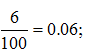n = Nwnber of years for which interest is calculated;

I = Amount of interest for the years at the given rate;

Pn = Principal with interest for the years added, or the total amount after the years.

Interest for n years, l = Prn

Total amount after n years, Pn = P + Prn = P (l + rn)

Interest rate r = l ÷ Pn

Number of years n = I ÷ Pn

Principal, or amount lent = I ÷ rn

The accurate interest for one day is 1/365 days of the interest for one year. Banks, however, customarily take the year as composed of 12 months of 30 days, making a total of 360 days.

Compound Interest

The following formulas are applicable when compound interest is to be computed, using the same notation as for simple interest, and assuming that the interest is compounded annually.

The total amount after n years, Pn = P (l + r)n

The principal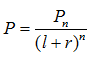The rate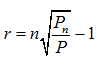The number of years during which the money is lent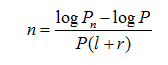Logarithms are especially useful in calculating compound interest. To find the total amount Pn of principal and interest after n years, the formula given can be transcribed as below:

log Pn = log P + n log (l + r)

However, logs are rarely used these days as scientific calculation functions are available even on simple calculators. The use of computer has also reduced the manual use of log tables for
calculations.

If the interest is payable q times a year, it will be computed q times during each year, or nq times during n years. The rate for each compounding will be (r ÷ q) if r is the annual rate. Hence, at the end of n years, the amount due will be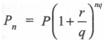Thus, if the term be 5 years, the interest payable quarterly, and the annual rate 6%, then n = 5, q = 4, r = 0.06; (r ÷ q) = 0.015, and nq = 20.

Important Formulas

The difference between simple interest and true discount on a sum of money is equal to the simple interest on the true discount for the given time at the given per cent rate.

(i) Sum = PV + TD (PV is 'Present Value')

(ii) Interest on sum = Interest on PV + Interest on TD or; = TD + Interest on TD

(iii) Interest on sum - TD = Interest on TD or; Banker's gain = Interest on TD.

True discount is mean proportional between the banker's gain and the true present value.

(i) PV : TD = Interest on PV : Interest on TD

(ii) PV : TD = TD : Banker's gain

(iii) (TD)2 = PV x Banker's gain